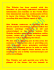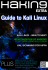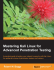# Questward

## Transcription

Questward
```I:
Ruff Country
Across the
Country with Kali
Part 3:
Qwestward
by Cletus Bianchi
andering souls d o n ' t do well i n sight of m o u n t a i n ranges or bends i n roads or rivers. No
m a t t e r how b e a u t i f u l or b o u n t i f u l the current locale, c u r i o s i t y always has us searching the h o r i z o n ,
stopping j u s t briefly before e x p l o r i n g farther. N i g h t f a l l is
the o n l y obstacle - not for fear of darkness, b u t because the
magnetic p u l l of the distance is d i m i n i s h e d .
W i t h six state flags on the h u n t i n g rig's bumper, f o u r
A m e r i c a n u p l a n d species i n Kali's nose, and a b u r g e o n i n g
c o l l e c t i o n of maps and web l i n k s , we were a n x i o u s to get
on the r o a d again. Kali's and m y enthusiasm for t h e h u n t 62
;
i n g a n d travel were equal, b u t I imagine m y m o t i v a t i o n s
were s l i g h t l y more c o m p l e x t h a n hers.
W h i l e I believed K a l i was capable of f i n d i n g any b i r d ,
anywhere, I wasn't quite sure I had the skill or fortitude to
m a k e i t happen. I l i k e d t h e travel. I l i k e d bragging about
t h e m u l t i p l e states and b i r d s . I l i k e d the research a n d the
new friendships. But t h e d i v e r s i t y of birds a n d h a b i t a t
was d a u n t i n g .
So, w h i l e i t was nice to d r e a m of an A m e r i c a n U p l a n d
G r a n d Slam and exciting to be on the road p u r s u i n g i t , I
c o u l d n ' t swear i t was an attainable, or even realistic, goal.
www.pointiiigdogjournal.com
THE
POINTING
DOG
JOURNAL
After our h u m b l i n g defeat i n the
Garibou-Targhee National Forest the
p r i o r season, I spent m u c h of the
s u m m e r w i t h Gene H i l l and George
B i r d Evans, s t u d y i n g up on ruffed
grouse. D o u b t f u l I c o u l d look the
p a r t of a proper grouse hunter, I d i d
hope to i n c o r p o r a t e the lingo i n t o
m y vocabulary. Folks i n South Texas
look at you f u n n y w h e n you p r o c l a i m
y o u "moved" a few doves on the last
pass t h r o u g h the sunflowers... u n s o phistieates!
W i t h our sights set o n M i c h i g a n ,
K a l i and I stopped i n San A n t o n i o to
p i c k up Sam Safir, a long-time f r i e n d
and h u n t i n g b u d d y ; and Cisco, h i s
new French B r i t t a n y . Sam was one of
m y mentors d u r i n g m y early gun dog
career; i n fact, shooting q u a i l over h i s
Brittanys i n s p i r e d me to get m y o w n
dog. Cisco is one of three French B r i t
acquisitions K a l i , i n t u r n , i n s p i r e d
amongst friends w h o had spent t i m e
w i t h her, w h e t h e r afield or on the
front porch. Somebody once referred
to these l i t t l e h u n t i n g companions as
"angels i n the house and devils i n the
field," and K a l i sold puppies based o n
b o t h characteristics.
(I knew K a l i was smart, b u t traveli n g w i t h Cisco b r o u g h t out her devious
side. She w o u l d ignore Cisco's every
attempt at play or f u n , regally t u r n i n g her head away or snapping at h i m ,
depending o n her m o o d . O n our way
to d i n n e r one n i g h t , we gave each dog
a rawhide on the t w o motel beds. K a l i
gnawed hers a b i t t h e n glanced over
where Cisco was i n chewing heaven.
She hopped over to his bed, slapped
her front paws d o w n i n a play bow, a n d
w h e n he j o y f u l l y leapt up, so excited
to f i n a l l y get to play, she snatched
Cisco's rawhide, j u m p e d back onto h e r
bed, and growled at h i m . Cisco h a d
the s t u n n e d a n d befuddled look of so
m a n y young h u m a n males desperate
to impress t h a t aloof girl.)
O u r first stop was i n n o r t h e r n
I l l i n o i s to h u n t a friend's family f a r m
for pheasants a n d b o b w h i t e . There
were some m e m o r a b l e points a n d reasonable success, b u t we were preoccupied w i t h the farmer's shop p a c k e d
w i t h b e a u t i f u l l y refurbished Oliver
tractors and a c o r n furnace - that's
how he spent t h e f r i g i d w i n t e r s . O n c e
September
/ October
2013
if.
I
The Au Sable State Forest in Michigan
provides
good ruffed grouse
woodcock
hunting
The overnight
snowfall
moved
timberdoodles
out and the ruffs into the heavier conifers for
cover.
After her airplane
trauma,
transportation
option.
mmm
www, polntinfidojiiournal.com
Kali
opted for
a slower
but more
and
the
comfortable
Stuart
first
Lowery, me, and Jim Funkhouser
Meams'quail.
walk
Blackhawk
again, the people a n d places along the road were as special
as the h u n t i n g .
Pre-trip research divulged a n u m b e r of t a n t a l i z i n g state
lands and public access areas between Niles and Battle Greek
i n southern M i c h i g a n , w i t h the p o t e n t i a l for pheasant, q u a i l ,
and ruffed grouse. We covered several properties i n a day
w i t h no success, so we headed farther n o r t h into notorious
" r u f f country."
The A u Sable State Forest covers a n i m m e n s e area i n
n o r t h - c e n t r a l M i c h i g a n , f r o m Lake H u r o n to Houghton Lake,
w i t h thousands of acres of hardwoods, "popple" (aspen), a n d
m i x e d conifers; countless r i p a r i a n areas; a n d interspersed
open fields. The Manistee National Forest continues westw a r d to Lake M i c h i g a n , p r o v i d i n g even more access.
O u r weather ranged f r o m dry, w a r m , a n d w i n d y to snowi n g i n a 4 8 - h o u r p e r i o d , so i t was a t o u g h game, especially
for novices. We m o v e d a good n u m b e r of birds, even saw
some, but drew no feathers. Since we chose to h u n t together
rather t h a n leap-frogging w i t h the r i g along clearcuts or
roads, we h u n t e d areas near road bends, so the w a l k b a c k
wasn't such a struggle. The adage about grouse cover slapp i n g you i n the face was p a i n f u l l y accurate.
We d i d l e a r n about woodcock a n d t h e i r preferred h a b i t a t ,
and both dogs were successful. Had I k n o w n then what I k n o w
now, I w o u l d have stayed up that n i g h t to watch the n i g h t
flight of the timberdoodles ahead of the m o r n i n g snowstorm!
There wasn't m u c h p o i n t "grousing" about the h u n t on the
way back to Texas, because we k n e w we h a d been close, t h e
research was productive, but the birds were as w i l y as the
timeless l i t e r a t u r e h a d foretold. A n d t h e staccato d r u m m i n g
of their escaping wings haunted m y dreams.
Agua es vida
Since s o u t h e r n New Mexico h a d enjoyed 125 percent of
n o r m a l p r e c i p i t a t i o n that year ( I ' m n o t k i d d i n g - weather
research is i m p o r t a n t ) , guess who suggested the family travel
64
Canyon
in the Gila National
Forest
where
Kali found
her
to the in-law's i n Las Cruces for T h a n k s g i v i n g ? K a l i was
disappointed she couldn't o c c u p y the entire back e n d of the
h u n t i n g r i g a n d was dubious about a l l the extra, non-essential items we packed, but ready to go nonetheless.
Ignore t h e T V ads - m y father-in-law Radon is t h e most
interesting m a n i n the w o r l d . He's witnessed nuclear
tests, m o d i f i e d weather, prospected for gold, a n d SGUBAdived i n caves, a l l w h i l e raising a daughter w i l l i n g to p u t
up w i t h me.
" B o o n d o c k i n g " is one of Radon's favorite pastimes, w h e t h er the excuse is mineralization or quail, so he was the perfect
w i n g m a n for o u r post-Thanksgiving t u r k e y adventure. Poor
Kali had a h a r d t i m e ignoring the huge covey of pet Gambel's
quail i n Radon's yard, but they presented a great o p p o r t u n i t y
for observation p r i o r to searching for t h e m i n the desert.
New Mexico's boot heel has t o n s of B L M and state lands
to access, b u t based u p o n r a i n f a l l totals, I was focused on
the L i t t l e H a t c h e t and A n i m a s M o u n t a i n s . I m i g h t have
made Radon a b i t nervous w h e n I p u l l e d over beside t w o
Border Patrol agents to ask if they'd seen birds. I figure they
k n o w e v e r y t h i n g that moves along the border, so w h y not?
They sent us farther west, so o n w a r d we traveled.
Had I k n o w n t h a t m o r n i n g how m a n y times K a l i a n d I
w o u l d cross t h e G o n t i n e n t a l Divide T r a i l , I may have given
it greater notice. I was focused o n a w i n d m i l l I c o u l d see
j u s t over the ridge. P u l l i n g i n t o its shadow, we saw Gambel's
q u a i l s c u r r y i n g away beneath t h e cholla cactus a n d creosote bushes. Scrambling a r o u n d , s l a m m i n g doors, a n d grabbing gear d i d n ' t settle the birds at a l l , and we spent an h o u r
unsuccessfully c o m b i n g the h i l l s i d e for the dozens of birds
we h a d j u s t seen.
inmpuBtt
THE
POINTING
DOG
JOURNAL
tiunterm
www.flushandpaint.cam
We c o n t i n u e d along the arroyo/road u n t i l we saw t h e next
w i n d m i l l u p against the west slope of I l a c h i t a Peak. More
cautious o n the approach this t i m e , we again found dozens of
birds loafing a r o u n d the water t r o u g h . W h i l e Radon watched
f r o m the r i g , K a l i and I eased o u r way toward the w i n d m i l l .
wwvv.puinrinj^dujijounial.com
btg trmtlitianal
Hunt Smart with Tough Bear
September
/ October
2013
vvww.poiiuiii^do^iouriiat.uoin
65
N G Sy 0 0 T£R
References
Michigan
• H u n t e r Access P r o g r a m , p r i v a t e l a n d s f o r p u b l i c
hunting www.michigan.gov/dnr/0,1607,7-15310363_ 10913_58762—,
00. html
• I n t e r a c t i v e m a p p i n g t o o l t o f i n d h u n t i n g access www.michigan.gov/dnr/0,1607,7-15310371J4793_55471—,
OO.html
New
Mexico
• D e m i n g : T h e Best W e s t e r n a l l o w s d o g s . T h e
G r a n d M o t o r I n n R e s t a u r a n t has h u g e b r e a k f a s t s
a n d h e l p f u l h u n t i n g t i p s . T h e r e ' s also g r e a t I t a l i a n a n d local w i n e s a t Raima's I t a l i a n G r i l l
• BLM maps a n d Gila N a t i o n a l Forest maps
a v a i l a b l e , b u t t h e Stilver City F o r e s t Service
o f f i c e is w o r t h a v i s i t - t h e y w e r e v e r y h e l p f u l .
http://plicmapcenter.
org.
Arizona
cover, Kali and I w o r k e d upslope to gain the
w i n d and t h e n d r o p p e d i n t o the b r u s h - c h o k e d
arroyo. I love a l l of t h e quails, especially the
Gambel's w i t h t h e i r j a u n t y t o p k n o t , so w h e n
K a l i p o i n t e d t h e m h u d d l e d against t h e cold
u n d e r some mesquite, I debated w h e t h e r to
d i s t u r b them. B u t one nervous b i r d broke, and
National
Forest in Arizona
is as difficult
as it is
beautit h e n another, a n d t h e n all were spewing f r o m
ful. Cactus, yucca, and mesquite
are hard on dog and hunters,
but
the bush like bees. T w o shots brought a beautihome to both scaled and Gambel's
quail.
ful pair to h a n d before we retreated to the t r u c k
and happily watched the birds regroup against
"Fellas...."
the worsening weather.
" I ' m t e l l i n g y o u , it's across the n e x t arroyo. We d i d n ' t
pass i t ! "
" H e y fellas, is this what
"Hey, fellas, is t h i s what we're l o o k i n g for r i g h t here?"
we're looldng f o r . . . ? "
O u t Stu's w i n d o w , at eye level f r o m the road c u t , a masThat Christmas I received Ben O. W i l l i a m s ' Hunting
the
sive covey of scalies was s t r e a m i n g by the rig, n o t 30 feet
Quails of North America
and finally resolved that Kali a n d
away. Brief pause... u t t e r chaos!
I w o u l d achieve t h e G r a n d Slam of A m e r i c a n q u a i l at a
W h e n we r e t u r n e d to the r i g an h o u r later, each w i t h
m i n i m u m . Lo a n d behold, Mearns' q u a i l , one of the m i s s p o i n t e d b i r d s i n the bag, I was t h a n k f u l the r a n c h r o a d
i n g three, live i n New Mexico. B r i m m i n g w i t h confidence
was seldom used. A l l five doors stood open. Shotgun shells
f r o m our recent success on desert q u a i l and rapidly e d u were spilled a l l a r o u n d . Maps h a d b l o w n off the dash and
cated on the b e a u t i f u l Mearns', I r e c r u i t e d E m i l Grow, a
i n t o the b r u s h . A n d all of o u r water bottles were s t i l l i n the
long-time f r i e n d a n d h u n t i n g c o m p a n i o n , and old college
t r u c k ! The Keystone Cops h u n t desert q u a i l . . . .
buddies S t u a r t L o w e r y and J i m F u n k h o u s e r to r e t u r n to
Despite l a c k of h y d r a t i o n . K a l i was on fire. She p i n n e d
New Mexico w i t h me.
those r u n n i n g b i r d s , scaled a n d Gambel's, against a r r o y o
O u r Gambel's covey wasn't at the first w i n d m i l l ; n o t h i n g at
edges for easy shots. She c i r c l e d wide and brought
birds
the second one, either. I felt a g r o w i n g sense of panic-unable
back to us. She scampered after cripples and p o i n t e d w i t h
to duplicate past success, missing t h e slam d u n k . W h i l e
birds i n her m o u t h . It was epic. A n d there were witnesses!
E m i l and I f u m b l e d w i t h maps, a r g u i n g over the location of
" Y o u guys javelina h u n t e r s ? "
the next w i n d m i l l , Stu rolled d o w n h i s backseat window.
" U h , fellas...."
B r e a k f a s t a n d blaze orange are a good c o m b i n a t i o n . As
No reply f r o m d r i v e r or navigator as the map argument
we ate, we were q u e r i e d by t w o o t h e r h u n t e r s , a n d w h e n
grew more intense.
we confessed we were after b i r d s , n o t peccary, one asked
66
www.pointingdoi^iournal.c'oni
THE
POINTING
DOG
JOURNAL
• Tombstone: The Tombstone M o t e l allows dogs,
is a c o o l a d o b e b u i l d i n g , a n d o n e b l o c k f r o m t h e
Crystal Palace! T h e r e a r e miles o f p u b l i c h u n t i n g
s u r r o u n d i n g T o m b s t o n e a n d all t h r e e q u a i l species a r e in t h e a r e a . P a t a g o n i a is a n o t h e r p o s sible s t o p p i n g s p o t .
i f I k n e w w h e r e t h e Big B u r r o M o u n t a i n s were. I d i d ,
t h a n k s to r e s e a r c h , a n d he suggested we l o o k for M e a r n s '
t h e r e . I n exchange, I d r e w h i m a m a p to o u r w i n d m i l l s .
We wolfed o u r b r e a k f a s t and set o u t for Silver C i t y , f o l l o w i n g t h e mojo despite e a r l i e r p l a n s .
Two hours later we were i n B l a c k h a w k Canyon i n t h e
Gila National Forest, h u n t i n g completely different h a b i t a t grassy savannah w i t h live oaks a n d cedar. K a l i locked u p b y
a low-hanging oak b r a n c h . We s u r r o u n d e d the tree, a n d t h e
Mearns' flushed i n all directions. O n c e again, I was blessed
w i t h a p a i r i n h a n d for the t a x i d e r m i s t , a n d we were t h a t
m u c h closer to t h e q u a i l slam.
The next day, we h u n t e d s o u t h e r n A r i z o n a for a l l t h r e e
species; and t h a t evening, we h u n t e d Tombstone for t h e
ghosts of Doc I l o l l i d a y and Big-Nose Kate.
(Here's a valuable h u n t i n g tip - keep an eye on the raptors!
D r i v i n g t h r o u g h the desert by the Peloncillo Mountains, Stu
noticed a hawk hovering over an arroyo. The hawk dove, the
q u a i l scattered, a n d we chased singles for an hour.)
By season's e n d , we were at eight u p l a n d species and
10 state flags. M y research techniques worked. The mojo
worked if we'd j u s t listen rather t h a n argue over maps. We
loved the West's tremendous access, spectacular beauty,
and u p l a n d b o u n t y . A n d the Q u a i l G r a n d
Slam was w i t h i n reach... after another
long s u m m e r !
Never d i d see a javelina.
• W i l c o x : Full services o n IH-10 a n d s u r r o u n d e d
b y g r e a t h u n t i n g - C o r o n a d o N a t i o n a l Forest
(Pinaleno M o u n t a i n s area), BLM (Peloncillo
M o u n t a i n s ) . Focus o n w a t e r ! W a t c h f o r r a p t o r s !
• Las C i e n e g a s N a t i o n a l C o n s e r v a t i o n A r e a is a n
a m a z i n g g r a s s l a n d in t h e m i d d l e o f t h e d e s e r t www.blm.gov/az/st/en/prog/blm_special_areas/
ncarea/lascienegas/hunting.html
Kali's first scaled quail
required
climbing
over 300feet
and took
its toll on us... a7id the birds.
The
Iiunting
rig is barely visible
behind
the yucca plant in the middle of the
photo. Thankfully,
it was
downhill!
```

### Cisco Recruitment Session* When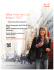### Pekiti Tirsia Global Grand Alliance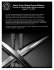### Brochure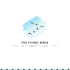### Archontophoenix alexandrae - foliage landscapes pty ltd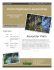### July-August 2014 Newsletter - Merrimack Valley Chapter of NAVHDA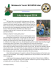### Ripley`s `14 Aquarium Slime Brochure.indd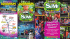### Paleornithology: Birds of the past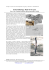### Muttonbird Panui (Flyer) 2014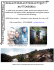### Upland Pie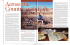development, so lodging may only be found in Dickinson. The area between Belfield and Bowman and north of Beach still holds good populations of pheasant and sharptails. favorite motel is the Edge o...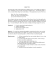### 101180_BIB Chinchilla A4.indd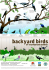### Jelovica, the shelter of silence Jelovica, the shelter of silence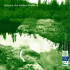### aigles royaux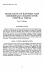### How to Perform your Rituals or Meditate by Hagur, Grand Hierophant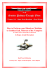### kali group study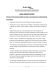### 132 - World`s No.1 Tantrik Astrologer